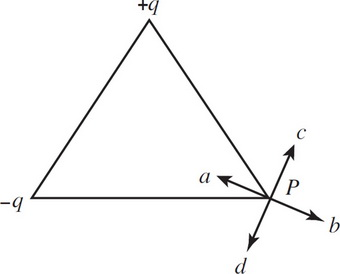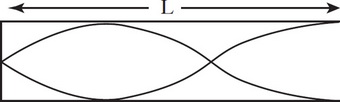# AP Physics 1 Practice Test 4

### Test Information10 questions18 minutes

1. A uniform bar is lying on a flat table. Besides the gravitational and normal forces (which cancel), the bar is acted upon by two other forces, F1 and F2, which are parallel to the surface of the table. If the net force on the rod is zero, then which one of the following is true?

2. An elevator is rising. In order to determine the tension in the cables pulling it, which values (for the elevator) would you need to know?

3.In the graph above, the instantaneous velocity at 9 seconds is closest to

4.Two charges are placed as shown at the vertices of an equilateral triangle. What is the direction of the electric field at point P?

5.If a charge of +q is placed at point P, the electric field at point P would

6.A tube with one end closed and one end open resonates for a wave with wavelength λa as shown. The next shorter wavelength at which resonance will occur is λb. The ratio of these two wavelengths λab is

7. A projectile of mass m is fired into the air at some angle θ. If its launch velocity is v0, which of the following expressions represents the object’s kinetic energy at the peak of its motion?

8. A man stands in an elevator as it begins to ascend. Does the normal force from the floor do work on the man?

9. An alarm whose frequency is 400 Hz is dropped out of a third-floor window. The student who drops it measures the frequency with a very sensitive oscilloscope. The measured frequency

10. A small cart of mass m is initially at rest. It collides elastically with a large cart of mass 4m and velocity v. The large cart loses half its kinetic energy to the little cart. The little cart now has a velocity of